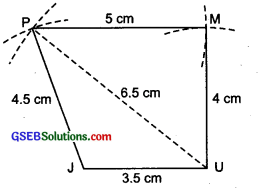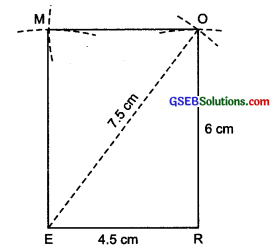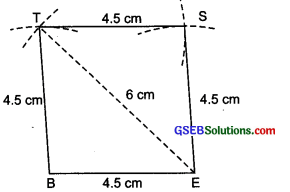# GSEB Solutions Class 8 Maths Chapter 4 Practical Geometry Ex 4.1

Gujarat Board GSEB Textbook Solutions Class 8 Maths Chapter 4 Practical Geometry Ex 4.1 Textbook Questions and Answers.

## Gujarat Board Textbook Solutions Class 8 Maths Chapter 4 Practical Geometry Ex 4.1

Question 1.
ABCD
AB = 4.5 cm
BC = 5.5 cm
CD = 4 cm
AC = 7 cmJUMP
JU = 3.5 cm
UM = 4 cm
MP = 5 cm
PJ = 4.5 cm
PU = 6.5 cm

(iii) Parallelogram
MORE
OR = 6 cm
BE = 4.5 cm
RE = 4.5 cm
ET = 6 cm
EO = 7.5 cm

(iv) Rhombus
BEST
BE = 4.5 cm
ET = 6 cm
Solution:
Note: Before making a fair construction for each of the above quadrilaterals, a rough sketch must be drawn and the given measurements be marked.(i) Steps of construction:I. Draw a line segment AB = 4.5 cm.
II. With centre at A and radius = 7 cm, draw an arc.
III. With centre at B and radius = 5.5 cm, draw another arc to intersect the previous arc at C.
IV. With centre at A and radius = 6 cm, draw an arc on the side opposite to that of B.
V. With centre at C and radius = 4 cm, draw another arc to intersect the previous arc at D.
VI. Join AC, BC, DA and DC. Thus, ABCD is the required quadrilateral

(ii) Steps of construction:I. Draw a line segment JU = 3.5 cm.
II. With centre at U and radius UP = 6.5 cm, draw an arc.
III. With centre at J and radius = 4.5 cm, draw another arc to intersect the previous arc at P.
IV. With centre at U and radius = 4 cm, draw an arc on the side opposite to that of J.
V With centre at P and radius = 4 cm, draw another arc intersecting the previous arc at M.
VI. Join PJ, PU, PM and UM. Thus, JUMP is the required quadrilateral.

(iii) Step of construction:I. Draw a line segment ER = 4.5 cm.
II. With centre at E and radius = 7.5 cm, draw an arc.
III. With centre at R and radius = 6 cm, draw another arc to intersect the previous arc at O.
IV. With centre at R and radius = 6 cm, draw an arc on the side opposite to R.
V. With centre at O and radius = 4.5 cm, draw another arc to intersect the previous arc at M.
VI. Join RO, EM, EO and MO.
Thus, EROM or MORE is the required parallelogram.(iv) Steps of construction:I. Draw a line segment BE = 4.5 cm.
II. With centre at E and radius = 6 cm, draw an arc.
III. With centre at B and radius = 4.5 cm, draw another arc to intersect the previous arc at T.
IV. Join TB and TE.
V. With centre at T and radius = 4.5 cm, draw an arc on the side opposite to that of B.
VI. With centre at E and radius = 4.5 cm, draw another arc to intersect the previous arc at S.
VII. Join ST and SE
Thus, BEST is the required rhombus.
Note: All the 4 sides of a rhombus are equal.